SELF 6 S.B. Karavashkin and O.N. KaravashkinaThis amount of problems is remained for today. And although we proved the principle, how the spiral dynamic field is formed, and this lifted some above problems of visualisation of stationary state of electron’s motion in atom, this principle as such also does not explain the no less important problem, how an orbital electron interacts with external fields that cause the electron’s excitation, as well as this issue was solved neither in Bohr’s theory nor in quantum-mechanical conceptions. Actually, in frames of Bohr’s formalism, the solving of problem was based on the general expression for the full energy of electron in its most general form ,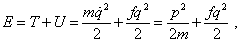(28)
 where in this case q meant the generalised coordinate of the point electron in the orbit that is thought as a linear oscillator, p is its pulse, and T and U are its kinetic and potential energy relatively. As the second modelling equation, there was used the postulate to select the quantum orbits that is the generalisation of Planck’s postulate; that Bohr used in his basic work “On the structure of atoms and molecules” . “In accordance with this last postulate, of all possible states of linear oscillator only those operate whose energy is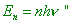(29)
 [9, p. 334]. Through the standard transformation and substitution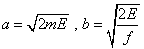(30)
 Shpolsky reduces (28) to the canonical form of ellipse,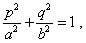(31)
 calculates the area of this geometric figure and yields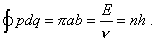(32)
 Then Shpolsky passes from generalised coordinates to those describing the electron’s orbital motion, taking such coordinate as the polar angle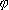that characterises the electron’s location in the circular orbit. With it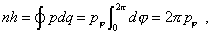(33)
 hence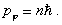(34)
 “This is the sought ‘rule of quantification’ of circular orbits: among all orbits possible in classical mechanics factually such work whose momentum is multiple of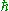. This last valueis, thus, the quantum unity of momentum” [9, p. 336]. With all beauty of this derivation, we see that, firstly, the statement of problem disregards the features of external force affection. As we will show here, this considerably changes the course of consideration. Secondly, the electron’s orbital motion is associated with a linear oscillator, while according to the statement of problem, the electron’s energy change has to change the orbital radius. But the Coulomb field is not linear, the more that according to (1), the radius of orbit depends on n as the square. Noting these factors, the statement of problem, as well as the phenomenology of excitation of orbital electron, is clearly insufficient to describe the processes. Below we will clarify the statement of problem and show considerable changes that are involved to the solution with this improvement. Given the problem as such is complicated, we will, as Bohr did, confine ourselves to the hydrogen atom with the circular orbit of electron and concentrate our attention only on the interaction with the external electric field. None the less, as we will see, even such narrow frame of study is able to reveal some basic features of the process, and the main, the cause, why both Bohr’s results and quantum-mechanical approach coincided in a so wonderful way.

Contents: / 1 / 2 / 3 / 4 / 5 / 6 / 7 / 8 / 9 / 10 / 11 / 12 / 13 / 14 / 15 /